# Random decimal picker thing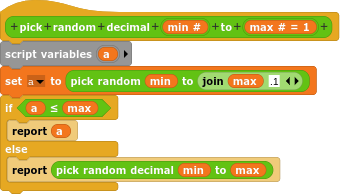The title is self-explanatory. It picks a random decimal between two numbers.

EDIT: It picks between two whole, rational integers.

EDIT 2: It should be less than, rather than less-than-or-equal-to.

(unrelated but i'm like really tired so sorry i haven't been as active recently? had a huge project do to irl soo)

You can just say "integers"; all integers are whole (another name for integer) and rational.

I'm curious why you used JOIN rather than +.

Oops. Forgot.

I don't know why I did that. Sorry if you were looking for a breakthrough insight or something.

right how dare he consider the possibility that you may have known something that he didn't instead of just assuming you had no idea what you were doing and correcting you!

No, just wondering. I have arguments with colleagues all the time about the number/numeral distinction in the context of conversion to and from binary, but here you're pretty much working entirely in the domain of numbers.

lol.

Good work

RecursivityWhy did you use recursivity instead of a "repeat while" loop?

This is my version: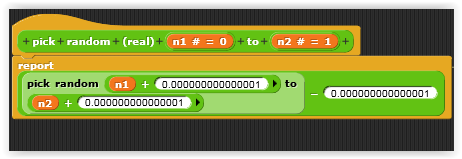edit: (the .0000000001 could be any decimal number...ex: .01)
Another idea: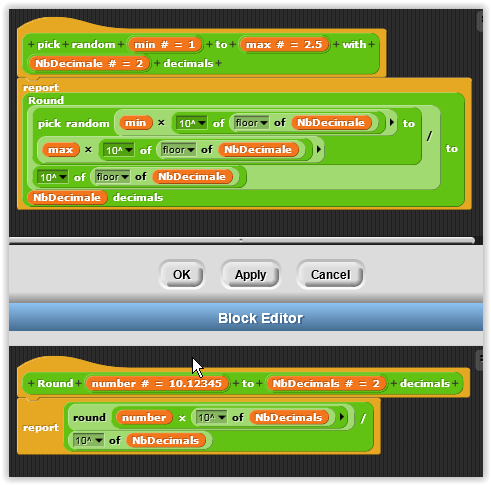(the round block is used in the pick random)

If we are going to optimise it then just need to add and subtract 0.1But I did like the original recursion conceptIn that case, 0.1 would be a better choice because it doesn't bring up the issue of floating point roundoff. But what if the input is something.9? Wouldn't you then get integer something+1?

Here's my logic:

I will demonstrate with integer but it's the same logic with decimals...

if you pick a random number between 1 and 3 you can get 1,2 or 3 right

if you pick a random number between (1+1) and (3+1) you can get 2,3 or 4 after you substract the result by 1 and you will get 1,2 or 3

if you add .1 instead of 1, the original random block will return a decimal:
if you pick a random number between (1+0.1) and (3+0.1) you can get 1.1 to 3.1 after you substract the result by 0.1 and you will get a decimal number between 1 and 3

if i want a decimal between 0.99999999 and 1.00000001, i will eventually get an integer: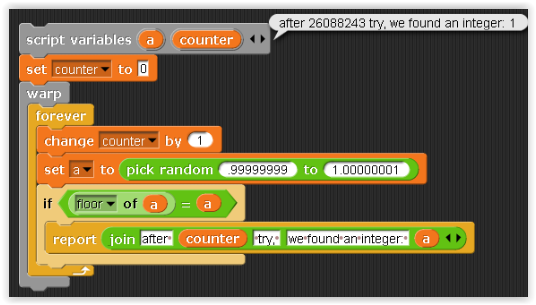hahaha after 26,000,000 times !

If you're programming a computerized casino, 1 error in 26M is too many. (And if you're programming the missiles carrying the nuclear bombs, it's WAY too many!)

Imho.

That's not an error... 1 (1.00000000000000000000) is a decimal

An integer is a decimal but a decimal isin't an integer

After exactly 26,088,243 times.

Let's see...(doing math)...the larger minus the smaller is 88,243...the smaller minus that is 25,911,757.

Are we talking CS-math or real-life-human-math? Because in CS-math, no, that's a floating-point number (between 0-1). In real-life-human-math, 1.0000(...) is an integer, because 1.0000(...) = 1.000 = 1.00 = 1.0 = 1. 1 is an integer.

Actually not CS-math but rather C-family-math.

I think we are talking at cross purposes. The error I had in mind isn't calling an integer a decimal; it's that your algorithm will (I think) give the wrong answer if the delta (0.1 or 0.001 or whatever) plus the decimal fraction part of the candidate random number (in 27.9 or 3.999 or whatever) add up exactly to 1. The integer part of the sum will then be one more than what it should be.

Touché !
I think i understand: If i add/substract 0.1, the decimal part of n1 AND n2 shouldn't be .9 because if there are, when i call the pick a random function with 2 integers, it return an integer instead of a real

example, i can't call my function with 1.9 and 2.9 (when i add/substract .1) (always return 1.9 or 2.9 instead of a real)

The old functionI modified the function slightly: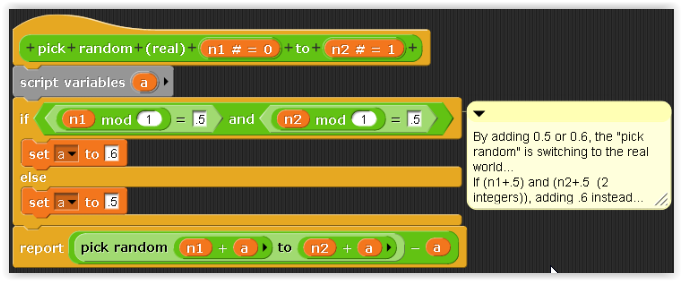(this is why i don't choose .1 for add/substract: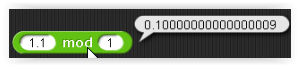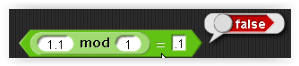)
(i don't have this problem with .5!)